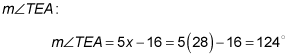##### Geometry: 1,001 Practice Problems For Dummies (+ Free Online Practice)Angles that form a linear pair combine to form a straight angle. (A straight angle measures 180 degrees.) The following practice questions ask you to solve problems based on linear pairs.

## Practice questions

In the following figure,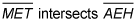at E. In the following questions, fill in the blank to make the statement true.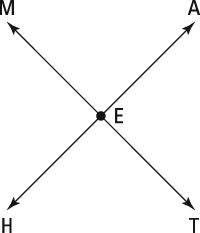1. If you know that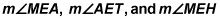are represented by 2a, 2a + b, and 3a – 20, respectively then b = ———?

2. If you know that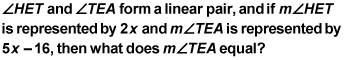1. 20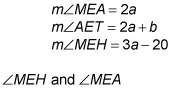form a linear pair, which means their sum is 180 degrees. Set up the following equation and solve for a: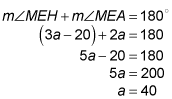Plug in the value of a to find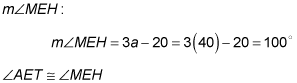because they’re vertical angles. Set them equal to each other, plug in the value of a, and solve for b: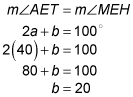2. 124 degrees

Angles that form a linear pair add up to 180 degrees. Set the sum of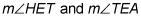equal to 180 and solve for x: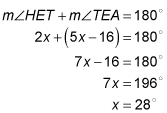Now plug in the value of x to solve for